# In Section 4.9 we showed how to use linear-programming duality theory to model the column and row players’ decision-making problems in a zero-sum two-person game

In Section 4.9 we showed how to use linear-programming duality theory to model the column and row players’ decision-making problems in a zero-sum two-person game. We would now like to exhibit a stronger connection between linear-programming duality and game theory. Consider the linear-programming dual problems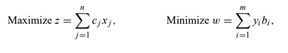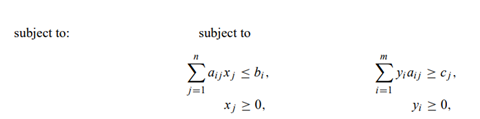where the inequalities apply for i = 1, 2, . . . , m and j = 1, 2, . . . , n; in addition, consider a zero-sum two-person game with the following payoff table: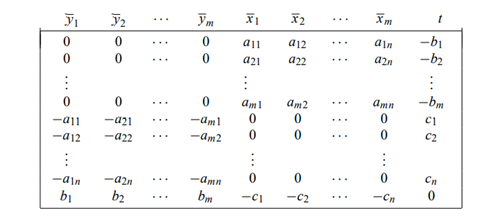The quantities Xj, Yi , and t shown above the table are the column players’ selection probabilities.

a) Is this game skew symmetric? What is the value of the game? (Refer to the previous exercise.)

b) Suppose that xj for j = 1, 2, . . . , n and yi for i = 1, 2, . . . , m solve the linear-programming primal and dual problems. Let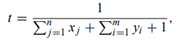let xj = txj for j = 1, 2, . . . , n, and let yi = tyi for i = 1, 2, . . . , m. Show that xj, yi , and t solve the given game.

c) Let xj for j = 1, 2, . . . , n, yi for i = 1, 2, . . . , m, and t solve the game, and suppose that t > 0. Show that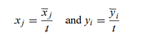solve the primal and dual linear programs. (See Hint.)

[Hint: Use the value of the game in parts (b) and (c), together with the conditions for strong duality in linear programming.]